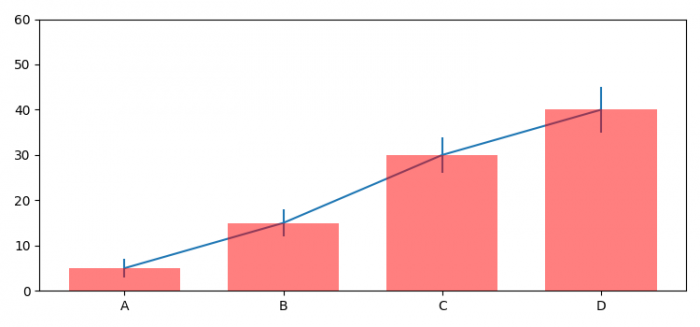# Indicating the statistically significant difference in bar graph (Matplotlib)

MatplotlibPythonData Visualization

To indicate the statistically significant difference in bar graph, we can take the following steps −

• Set the figure size and adjust the padding between and around the subplots.
• Create means, std, index, width and labels data points.
• Create a figure and a set of subplots using subplots() method.
• Make a bar plot using bar() method.
• Plot Y versus X as lines and/or markers with attached errorbars.Scale the Y-axis.
• Get or set the current tick locations and labels of the X-axis.
• To display the figure, use show() method.

## Example

import numpy as np
import matplotlib.pyplot as plt
plt.rcParams["figure.figsize"] = [7.50, 3.50]
plt.rcParams["figure.autolayout"] = True

means = (5, 15, 30, 40)
std = (2, 3, 4, 5)
index = np.arange(4)
width = 0.7
labels = ('A', 'B', 'C', 'D')

fig, ax = plt.subplots()
ax.p1 = plt.bar(index, means, width=width, color="red",
linewidth=2, zorder=5, alpha=0.5)
ax.errs = plt.errorbar(index, means, yerr=std)

plt.ylim(ymax=60)
plt.xticks(index, labels, color='k')

plt.show()

## Output